# Imputation of Financial Time Series

### 2020-07-11

This vignette illustrates the usage of the package imputeFin for imputation of missing values and detection/removal of outliers in time series that follow a random walk or an autoregressive (AR) model. As a side result, the parameters of the model are estimated from the incomplete time series.

# Installation

The package can be installed from CRAN or GitHub:

# install stable version from CRAN
install.packages("imputeFin")

# install development version from GitHub
devtools::install_github("dppalomar/imputeFin")

To get help:

library(imputeFin)
help(package = "imputeFin")
?impute_AR1_Gaussian
vignette("ImputeFinancialTimeSeries", package = "imputeFin")
RShowDoc("ImputeFinancialTimeSeries", package = "imputeFin")

To cite package imputeFin or the base reference in publications:

citation("imputeFin")

# Quick Start

Let’s load some time series data with missing values for illustration purposes:

library(xts)
library(imputeFin)
data(ts_AR1_t)
names(ts_AR1_t)
#>  "y_missing" "phi0"      "phi1"      "sigma2"    "nu"

We can then impute one of the time series and plot it:

y_missing      <- ts_AR1_t$y_missing[, 3] y_missing <- 2*y_missing # create an outlier plot_imputed(y_missing, title = "Original time series with missing values and one outlier")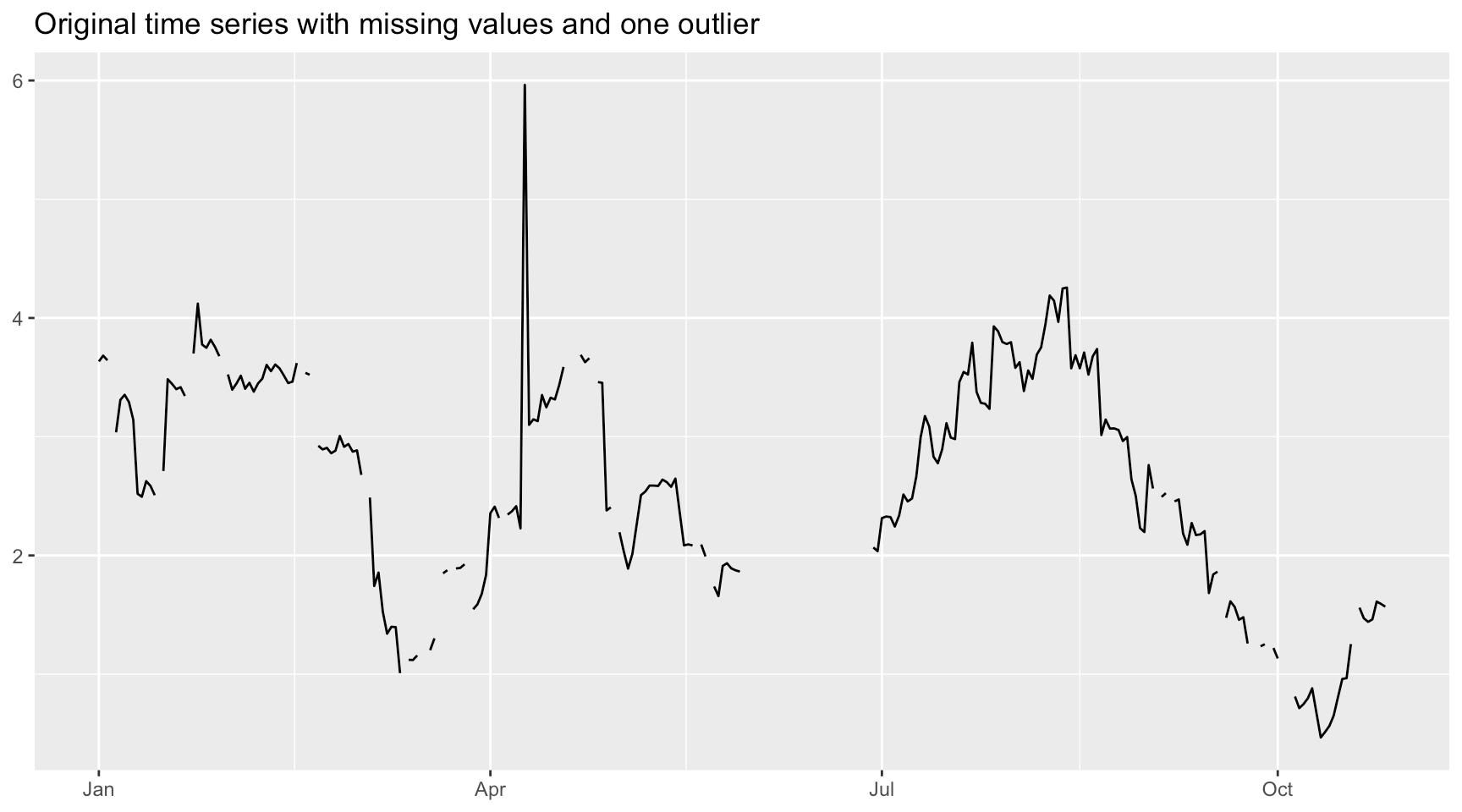y_imputed <- impute_AR1_t(y_missing, remove_outliers = TRUE) #> var c: 60 missing values imputed and 1 outliers detected and corrected. plot_imputed(y_imputed)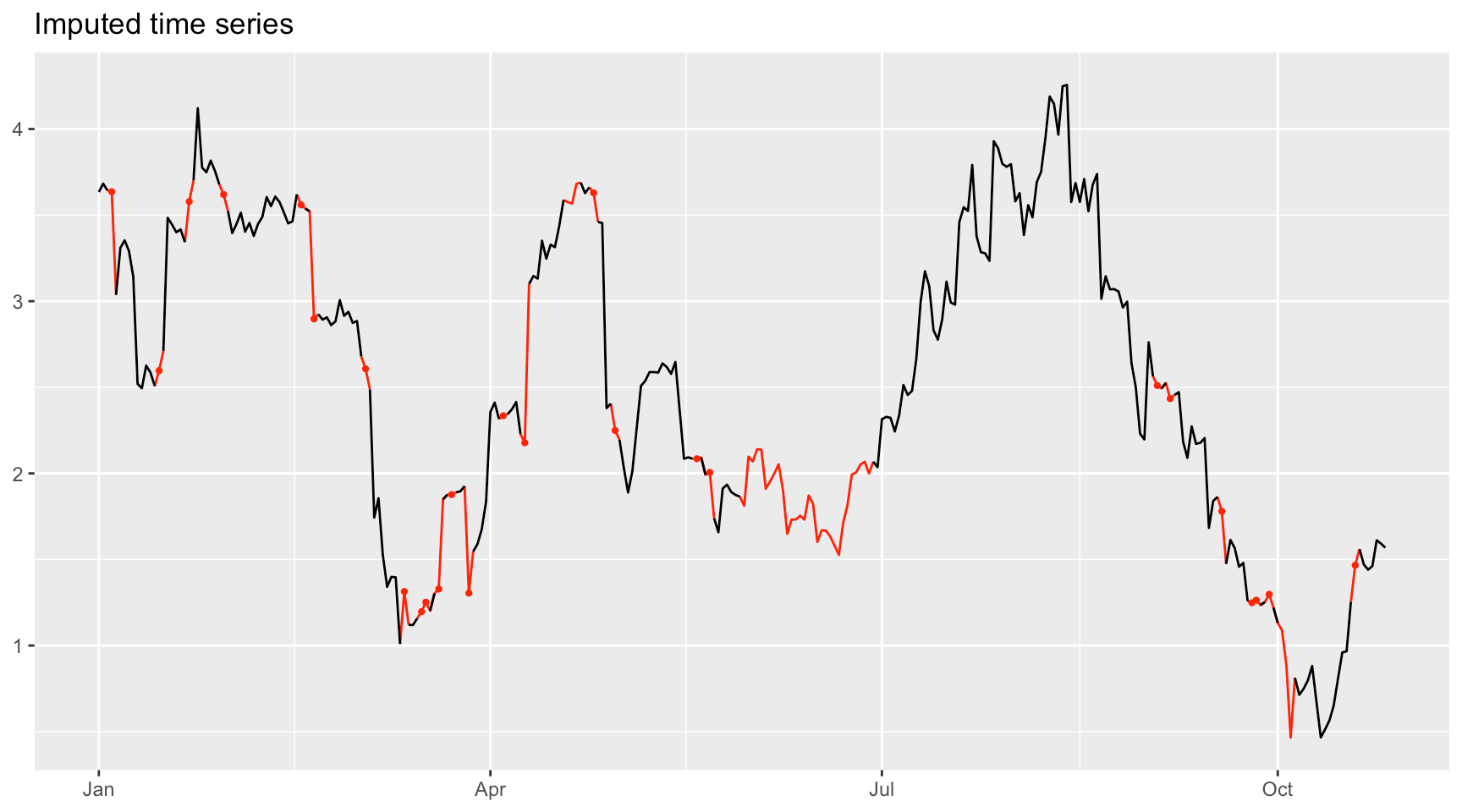# Usage of the package This package can be used to impute missing values in time series and detect/remove outliers that follow a random walk or an AR(1) model. Besides, it can be used to estimate the model parameters of the models from incomplete time series with missing values. ## Datasets To use this package, the time series object with missing values should be coercible to either a numeric vector or numeric matrix (e.g., matrix, zoo, or xts) with missing values denoted by NA. For convenience, the package contains two time series objects with missing values: • Gaussian AR(1) time series: ts_AR1_Gaussian is a list containing a time series with missing values y_missing (with three columns) generated from an AR(1) model with Gaussian distributed innovations, and the parameters of the model phi0, phi1, and sigma2; • Student’s $$t$$ AR(1) time series: ts_AR1_t is a list containing a time series with missing values y_missing (with three columns) generated from an AR(1) model with Student’s $$t$$ distributed innovations, and the parameters of the model phi0, phi1, sigma2, and nu. library(imputeFin) data(ts_AR1_Gaussian) data(ts_AR1_t) names(ts_AR1_t) #>  "y_missing" "phi0" "phi1" "sigma2" "nu" ## Fitting a Gaussian AR(1) model We start with the function fit_AR1_Gaussian() to fit a univariate Gaussian AR(1) model and estimate the parameters: y_missing <- ts_AR1_Gaussian$y_missing[, 2]  # choose second column / time series
fitted <- fit_AR1_Gaussian(y_missing)
#> var b: 30 inner missing values and 0 outliers detected.
fitted
#> $phi0 #>  1.063603 #> #>$phi1
#>  0.4674481
#>
#> $sigma2 #>  0.009669752 #> #>$index_miss
#>     3   4  17  21  79  97 102 130 133 141 145 151 154 168 190 198 201 204 205
#>  218 222 236 237 238 270 273 275 295 297 299
#>
#> $index_outliers #> NULL If instead we want to fit a random walk model, which means that phi1 = 1, then we can set the argument random_walk = TRUE (similarly, if we want to force a zero mean, then we can set zero_mean = TRUE): fitted <- fit_AR1_Gaussian(y_missing, random_walk = TRUE) #> var b: 30 inner missing values and 0 outliers detected. fitted #>$phi0
#>  0.001318797
#>
#> $phi1 #>  1 #> #>$sigma2
#>  0.01263339
#>
#> $index_miss #>  3 4 17 21 79 97 102 130 133 141 145 151 154 168 190 198 201 204 205 #>  218 222 236 237 238 270 273 275 295 297 299 #> #>$index_outliers
#> NULL

For multivariate time series, the function fit_AR1_Gaussian() can still be used but it simply works on each univariate time series individually (thus no multivariate fitting, just univariate fitting). In the following example, the object y_missing contains three different time series named ‘a’, ‘b’, and ‘c’. The function fit_AR1_Gaussian() fits each time series separately and the returned value is a list consisting of the estimation results for each time series and additional elements that combine the estimated values in a convenient vector form:

y_missing <- ts_AR1_Gaussian$y_missing fitted <- fit_AR1_Gaussian(y_missing) #> var a: 30 inner missing values and 0 outliers detected. #> var b: 30 inner missing values and 0 outliers detected. #> var c: 57 inner missing values and 0 outliers detected. names(fitted) #>  "var a" "var b" "var c" "phi0_vct" "phi1_vct" #>  "sigma2_vct" fitted$a
#> NULL
fitted$phi0_vct #> var a var b var c #> 1.034113 1.063603 1.011861 ## Fitting a Student’s t AR(1) model The function fit_AR1_t() works similarly to fit_AR1_Gaussian() but assuming that the residuals follow a Student’s $$t$$ distribution: y_missing <- ts_AR1_t$y_missing[, 2]
fitted <- fit_AR1_t(y_missing)
#> var b: 30 inner missing values and 0 outliers detected.
fitted
#> $phi0 #>  0.04725166 #> #>$phi1
#>  0.9827364
#>
#> $sigma2 #>  0.007565988 #> #>$nu
#>  1.764065
#>
#> $index_miss #>  4 15 22 30 48 51 63 72 76 77 80 83 87 95 110 112 116 121 140 #>  143 153 247 250 262 269 270 273 276 278 293 #> #>$index_outliers
#> NULL

It is important to note the argument fast_and_heuristic, which indicates whether a heuristic but fast method is to be used to estimate the parameters (by default, it is TRUE).

## Imputation of missing values from Gaussian AR(1) model

We now show how to use the function impute_AR1_Gaussian() to impute the missing values in the time series based on the Gaussian AR(1) model, and how to conveniently plot the imputed time series with the function plot_imputed():

y_missing <- ts_AR1_Gaussian$y_missing[, 1] y_imputed <- impute_AR1_Gaussian(y_missing) #> var a: 30 missing values imputed and 0 outliers detected and corrected. plot_imputed(y_imputed)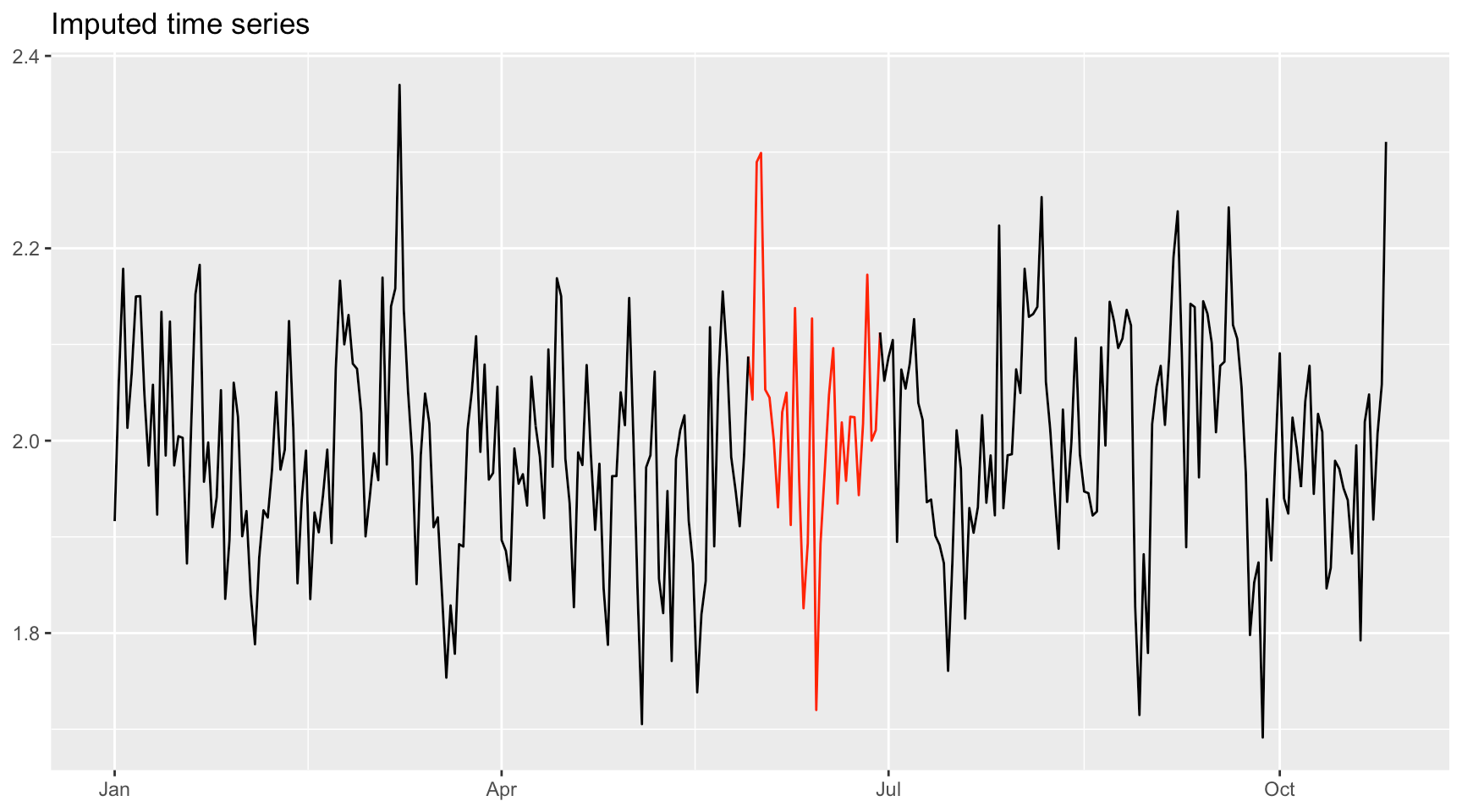The function impute_AR1_Gaussian() first fits the Gaussian AR(1) model to the incomplete time series data with missing values, and then imputes the missing values by drawing samples from the conditional distribution of the missing values given the observed data based on the estimated Gaussian AR(1) model. By default, the number of imputations is 1 (n_samples = 1), and the function impute_AR1_Gaussian() returns an imputed time series of the same class and dimensions as the input data but with one new attribute recording the locations of the missing values (the function plot_imputed() makes use of such information to indicate the imputed values). If multiple imputations are desired, simply set the argument n_samples to the desired number. Then the function will return a list consisting of each imputed time series: res <- impute_AR1_Gaussian(y_missing, n_samples = 3) #> var a: 30 missing values imputed and 0 outliers detected and corrected. names(res) #>  "y_imputed.1" "y_imputed.2" "y_imputed.3" In addition to the imputed time series, the function can return the estimated parameters of the model by setting the argument return_estimates = TRUE (by default, it is FALSE): res <- impute_AR1_Gaussian(y_missing, n_samples = 3, return_estimates = TRUE) #> var a: 30 missing values imputed and 0 outliers detected and corrected. names(res) #>  "y_imputed.1" "y_imputed.2" "y_imputed.3" "phi0" "phi1" #>  "sigma2" ## Imputation of missing values from Student’s t AR(1) model The function impute_AR1_t() works similarly to impute_AR1_Gaussian() but assuming that the residuals follow a Student’s $$t$$ distribution: y_missing <- ts_AR1_t$y_missing[, 1]
res <- impute_AR1_t(y_missing, n_samples = 3, return_estimates = TRUE)
#> var a: 30 missing values imputed and 0 outliers detected and corrected.
names(res)
#>  "y_imputed.1" "y_imputed.2" "y_imputed.3" "phi0"        "phi1"
#>  "sigma2"      "nu"
plot_imputed(res$y_imputed.1)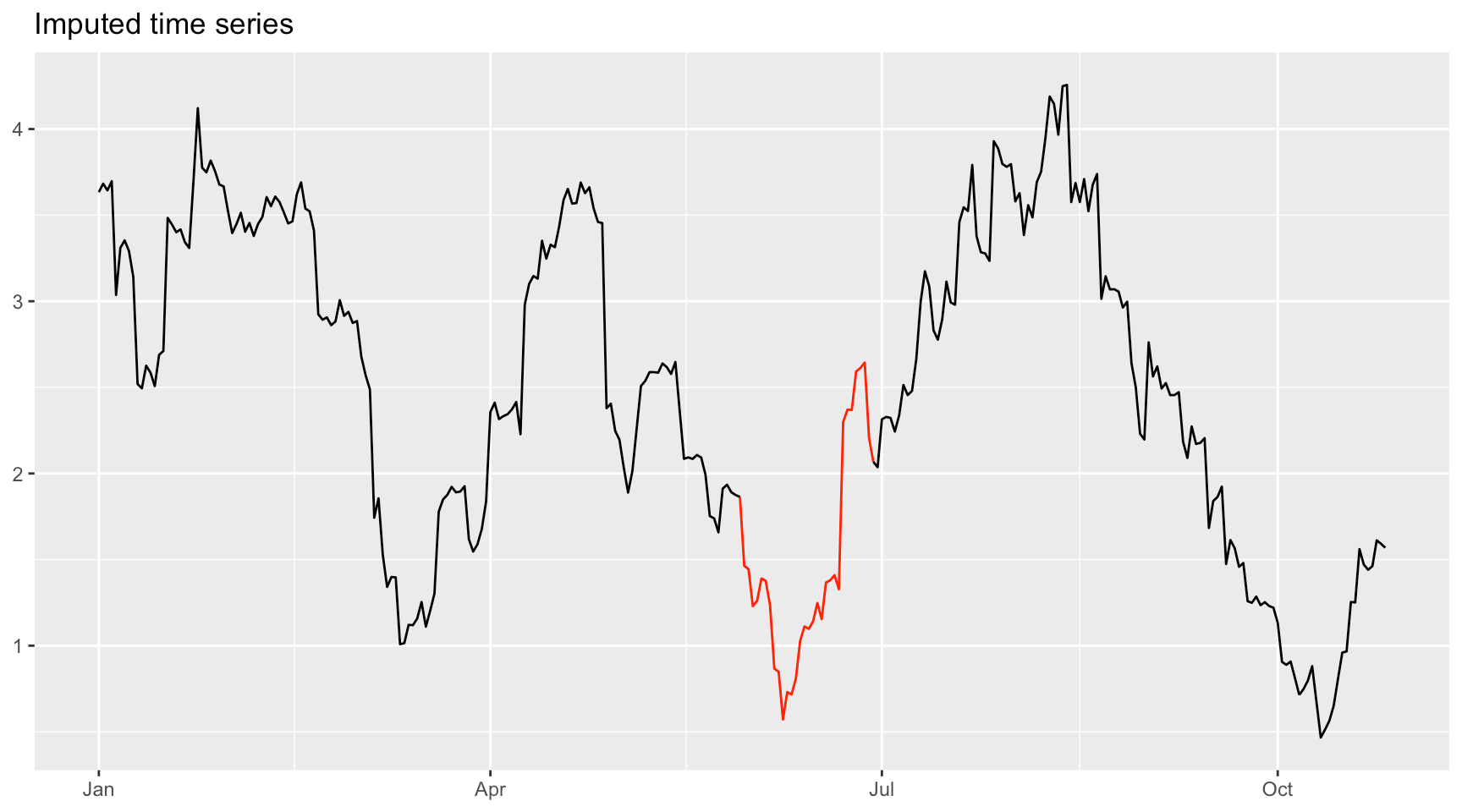## Removing outliers In addition to imputing missing values, the functions also are equipped to detect and remove (and then impute) outliers. This is easily accomplished with the argument remove_outliers = TRUE: y_missing <- ts_AR1_t$y_missing[, 3]
y_missing <- 2*y_missing  # create an outlier
plot_imputed(y_missing,
title = "Original time series with missing values and one outlier")y_imputed <- impute_AR1_t(y_missing, remove_outliers = TRUE, outlier_prob_th = 1e-3)
#> var c: 60 missing values imputed and 1 outliers detected and corrected.
plot_imputed(y_imputed)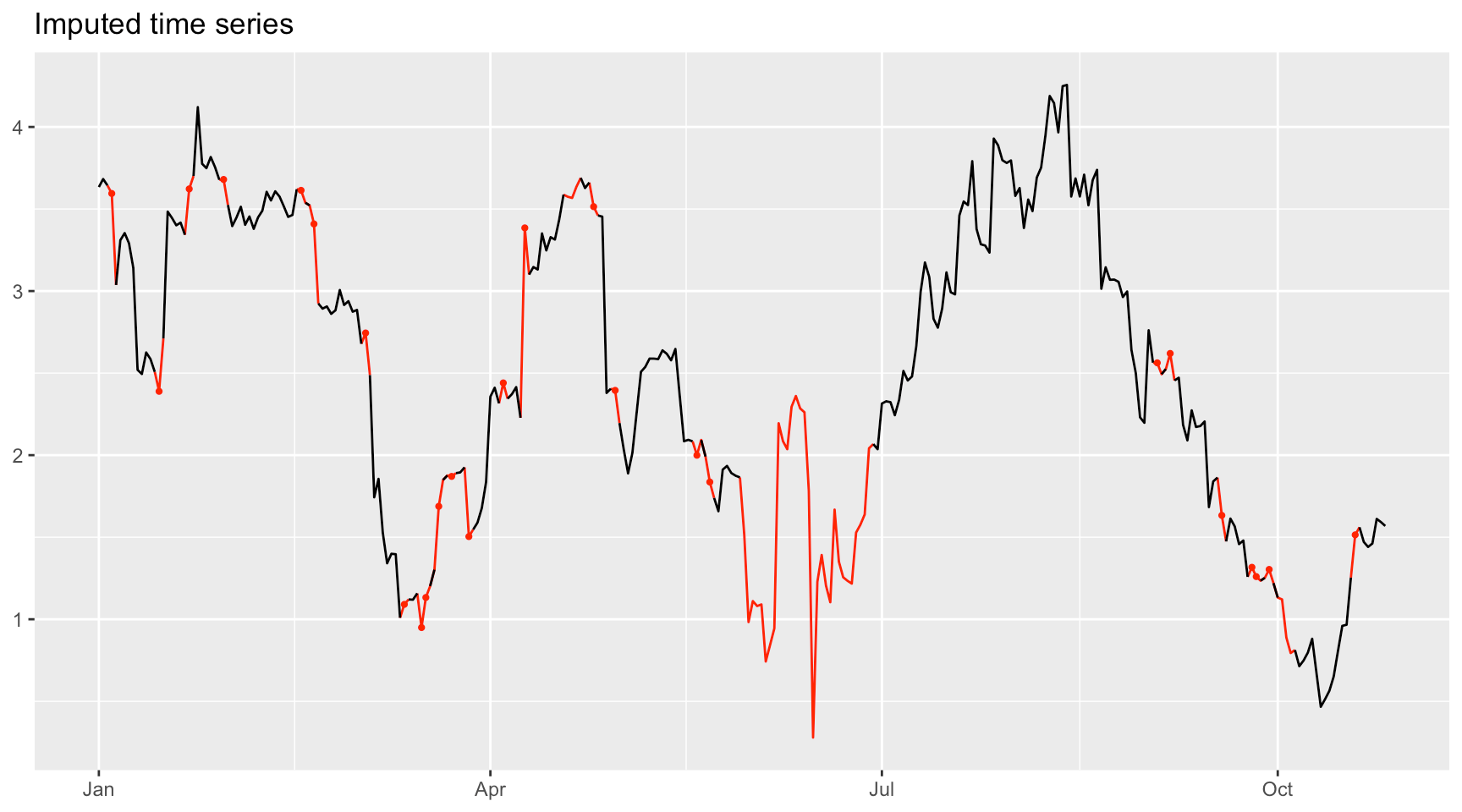Note, however, that in the current version it cannot fix price jumps arising from stock splits.

# Comparison with other packages

We compare our package with the existing packages zoo and imputeTS. We first download the adjusted prices of the S&P 500 index from 2012-01-01 to 2015-07-08, compute the log-prices, and intentionally delete some of them for illustrative purposes.

# download data
library(quantmod)
y_orig <- log(Ad(getSymbols("^GSPC", from = "2012-01-01", to = "2015-07-08",
auto.assign = FALSE)))
T <- nrow(y_orig)

# introduce 20% of missing values artificially
miss_pct <- 0.2
T_miss <- floor(miss_pct*T)
index_miss <- round(T/2) + 1:T_miss
attr(y_orig, "index_miss") <- index_miss
y_missing <- y_orig
y_missing[index_miss] <- NA

Now we plot the imputed time series obtained by functions in existing packages zoo and imputeTS. Basically, all these interpolation methods look artificial and destroy the time series statistics:

# impute using packages zoo and imputeTS
library(zoo)
library(imputeTS)
y_imputed_locf   <- zoo::na.locf(y_missing)
y_imputed_linear <- zoo::na.approx(y_missing)
y_imputed_ma     <- imputeTS::na_ma(y_missing)
y_imputed_spline <- imputeTS::na_interpolation(y_missing, "spline")
y_imputed_stine  <- imputeTS::na_interpolation(y_missing, "stine")
y_imputed_kalman <- imputeTS::na_kalman(y_missing)

# plots
p1 <- plot_imputed(y_orig, title = "Original")
p2 <- plot_imputed(y_imputed_locf, title = "Imputation with LOCF")
p3 <- plot_imputed(y_imputed_ma, title = "Imputation with MA")
p4 <- plot_imputed(y_imputed_linear, title = "Imputation with linear interpolation")
p5 <- plot_imputed(y_imputed_spline, title = "Imputation with spline interpolation")
p6 <- plot_imputed(y_imputed_stine, title = "Imputation with Stineman interpolation")
gridExtra::grid.arrange(p1, p2, p3, p4, p5, p6, ncol = 2)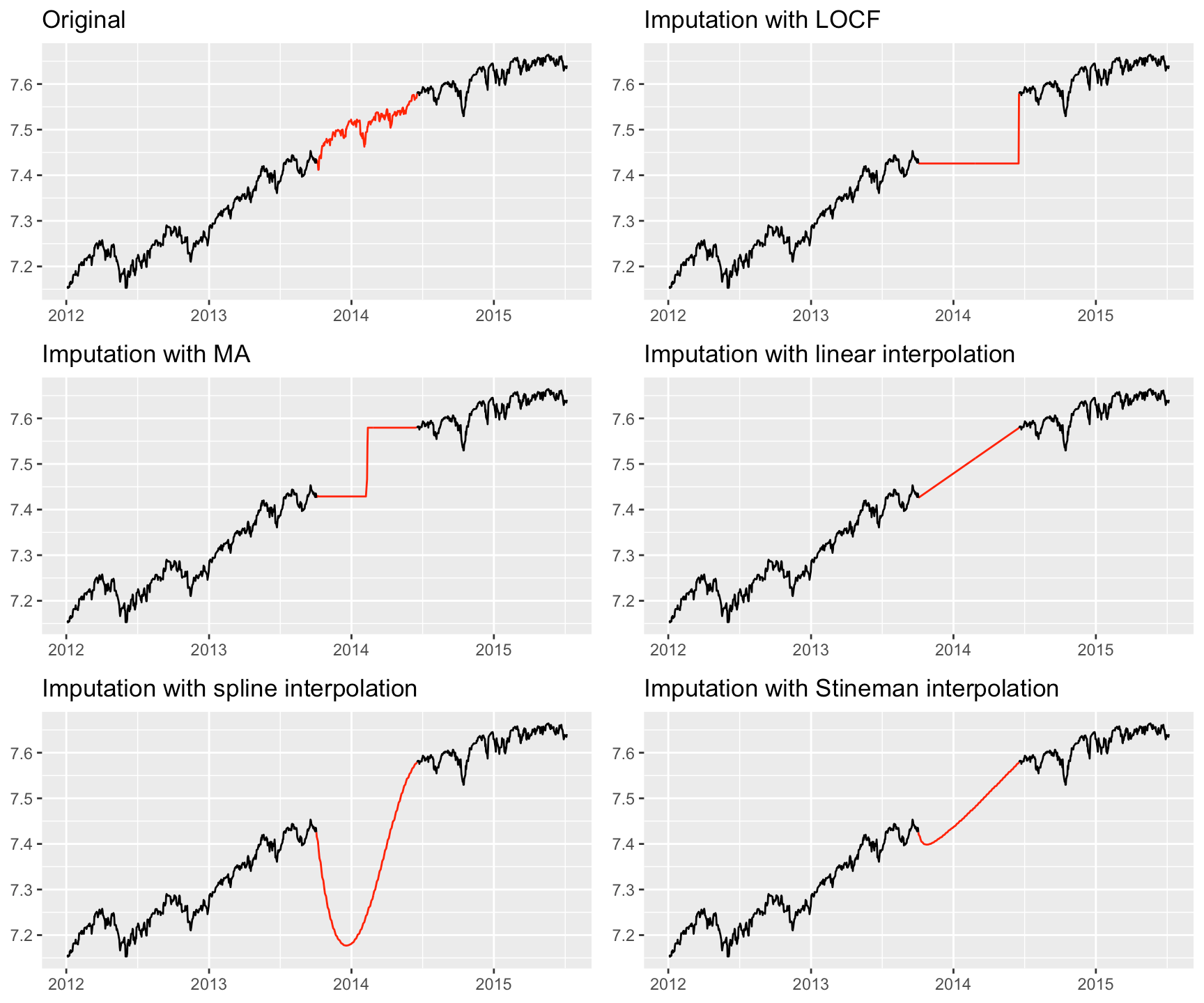On the other hand, the function impute_AR1_t() from the package imputeFin preserves the time series statistics and looks realistic:

# impute using  package imputeFin
library(imputeFin)
res <- impute_AR1_t(y_missing, n_samples = 3)

# plots
p1 <- plot_imputed(y_orig, title = "Original")
p2 <- plot_imputed(res$y_imputed.1, title = "Imputation 1") p3 <- plot_imputed(res$y_imputed.2, title = "Imputation 2")
p4 <- plot_imputed(res\$y_imputed.3, title = "Imputation 3")
gridExtra::grid.arrange(p1, p2, p3, p4, ncol = 2)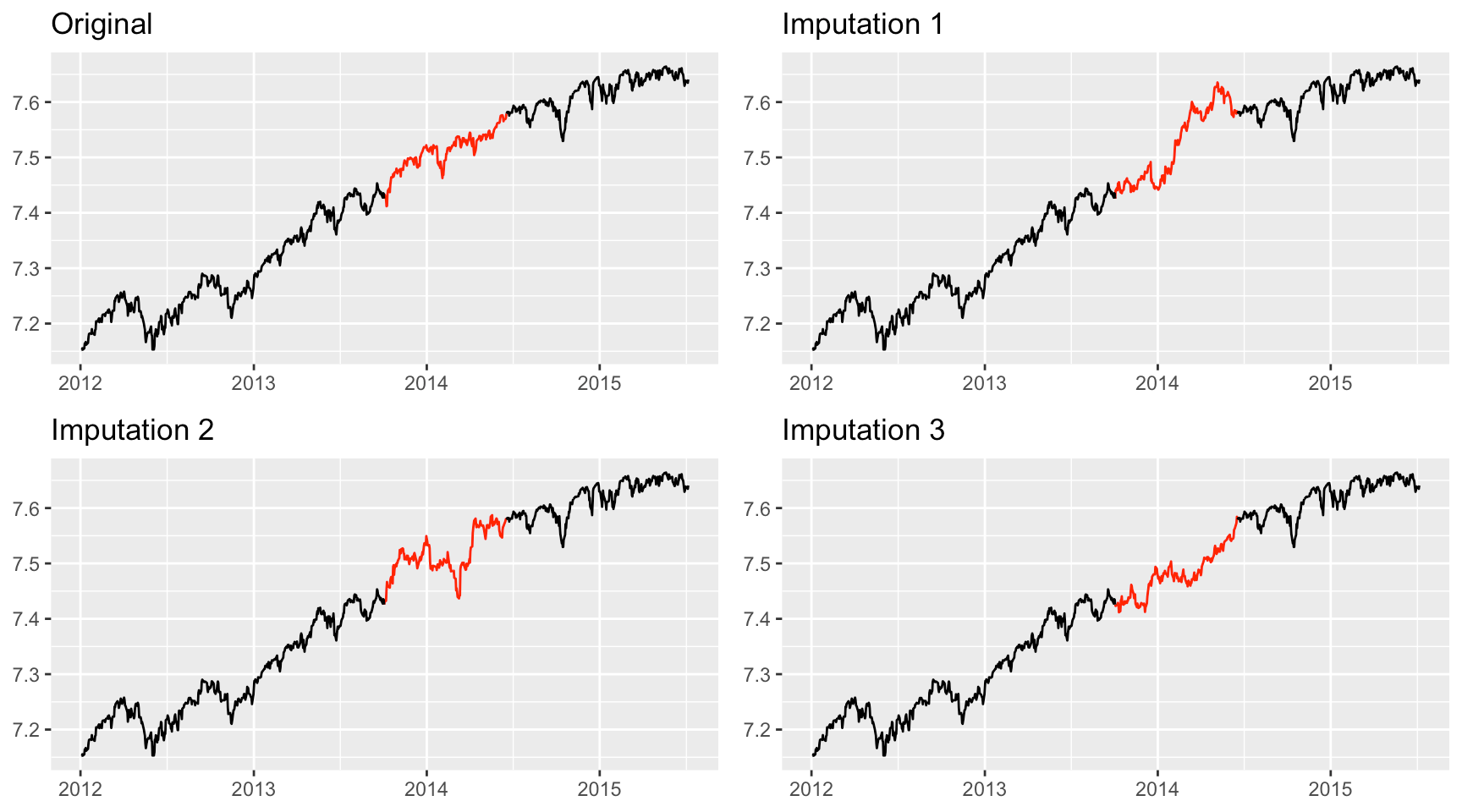# Algorithms

## Parameter estimation

The parameter estimation for the AR(1) models with Gaussian and Student’s $$t$$ distributed innovations are based on the maximum likelihood estimation (MLE) given the observed data. Suppose we have a univariate time series $$y_{1}$$, $$y_{2}$$,$$\ldots$$, $$y_{T}$$ from the Gaussian AR($$1$$) model $\begin{equation} y_{t}=\varphi_{0}+\varphi_{1}y_{t-1}+\varepsilon_{t},\label{eq:ar(1) model} \end{equation}$ where $$\varepsilon_{t}\overset{i.i.d.}{\sim}\mathcal{N}\left(0,\sigma^{2}\right)$$. Some values are missing during the collection, and we denote the missing values by $$\mathbf{y}_{\mathsf{miss}}$$. Then MLE problem for the parameters of the Gaussian AR(1) model takes the form:

\begin{equation} \begin{aligned}\mathsf{\underset{\varphi_{0},\varphi_{1},\sigma^{2}}{maximize}} & \thinspace\thinspace\thinspace\log\left(\int\prod_{t=2}^{T}f_{G}\left(y_{t};\varphi_{0}+\varphi_{1}y_{t-1},\sigma^{2}\right)\mathsf{d}\mathbf{y}_{\mathsf{miss}}\right),\end{aligned} \end{equation} where $$f_{G}\left(\cdot\right)$$ denotes the probability density function (pdf) of a Gaussian distribution.

For Student’s $$t$$ AR(1) model with $$\varepsilon_{t}\overset{i.i.d.}{\sim}t\left(0,\sigma^{2},\nu\right)$$, the MLE problem for the parameters takes the form: \begin{equation} \begin{aligned}\mathsf{\underset{\varphi_{0},\varphi_{1},\sigma^{2},\nu>0}{maximize}} & \thinspace\thinspace\thinspace\log\left(\int\prod_{t=2}^{T}f_{t}\left(y_{t};\varphi_{0}+\varphi_{1}y_{t-1},\sigma^{2},\nu\right)\mathsf{d}\mathbf{y}_{\mathsf{miss}}\right),\end{aligned} \label{eq:problem formulation-2} \end{equation} where $$f_{t}\left(\cdot\right)$$ denotes the probability density function (pdf) of a Gaussian distribution.

The objective functions in the above optimization problems are very complicated, and there are no closed-form solutions for them. Thus, it is necessary to resort to the expectation-maximization (EM) framework to derive efficient iterative algorithms to solve these MLE problems. An EM agorithm was developed to estimate the parameters for the Gaussian case . The stochastic version of the EM algorithm has been derived to deal with the Student’s $$t$$ case .

## Imputation

Given the conditional distribution $$p\left(\mathbf{y}_{\mathsf{miss}}|\mathbf{y}_{\mathsf{obs}}\right)$$ with $$\mathbf{y}_{\mathsf{obs}}$$ being the observed values, it is trivial to impute the missing values by randomly drawing realizations from $$p\left(\mathbf{y}_{\mathsf{miss}}|\mathbf{y}_{\mathsf{obs}}\right)$$. However, in our case, we do not have the conditional distribution $$p\left(\mathbf{y}_{\mathsf{miss}}|\mathbf{y}_{\mathsf{obs}}\right)$$ in closed form. An improper way of imputing (which is acceptable in many cases with small percentage of missing values) is with $$p\left(\mathbf{y}_{\mathsf{miss}}|\mathbf{y}_{\mathsf{obs}},\boldsymbol{\theta}^{\mathsf{ML}}\right)$$, where $$\boldsymbol{\theta}^{\mathsf{ML}}$$ is the MLE result for the model parameter. Due to the complexity of the conditional distribution $$p\left(\mathbf{y}_{\mathsf{miss}}|\mathbf{y}_{\mathsf{obs}},\boldsymbol{\theta}^{\mathsf{ML}}\right)$$, we cannot sample from it direcly, and a Gibbs sampling scheme is designed to draw realizations.